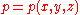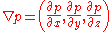xEncyclopedia
In atmospheric sciences the pressure gradient (typically of air
Earth's atmosphere
The atmosphere of Earth is a layer of gases surrounding the planet Earth that is retained by Earth's gravity. The atmosphere protects life on Earth by absorbing ultraviolet solar radiation, warming the surface through heat retention , and reducing temperature extremes between day and night...

, more generally of any fluid
Fluid
In physics, a fluid is a substance that continually deforms under an applied shear stress. Fluids are a subset of the phases of matter and include liquids, gases, plasmas and, to some extent, plastic solids....

) is a physical quantity that describes in which direction and at what rate the pressure
Pressure
Pressure is the force per unit area applied in a direction perpendicular to the surface of an object. Gauge pressure is the pressure relative to the local atmospheric or ambient pressure.- Definition :...

changes the most rapidly around a particular location. The pressure gradient is a dimensional quantity expressed in units
Units of measurement
A unit of measurement is a definite magnitude of a physical quantity, defined and adopted by convention and/or by law, that is used as a standard for measurement of the same physical quantity. Any other value of the physical quantity can be expressed as a simple multiple of the unit of...

of pressure per unit length. The SI
International System of Units
The International System of Units is the modern form of the metric system and is generally a system of units of measurement devised around seven base units and the convenience of the number ten. The older metric system included several groups of units...

unit is pascal
Pascal (unit)
The pascal is the SI derived unit of pressure, internal pressure, stress, Young's modulus and tensile strength, named after the French mathematician, physicist, inventor, writer, and philosopher Blaise Pascal. It is a measure of force per unit area, defined as one newton per square metre...

per metre
Metre
The metre , symbol m, is the base unit of length in the International System of Units . Originally intended to be one ten-millionth of the distance from the Earth's equator to the North Pole , its definition has been periodically refined to reflect growing knowledge of metrology...

(Pa/m).

## Mathematical description

Assuming that the pressure p is an intensive quantity, i.e., a single-valued, continuous
Continuous function
In mathematics, a continuous function is a function for which, intuitively, "small" changes in the input result in "small" changes in the output. Otherwise, a function is said to be "discontinuous". A continuous function with a continuous inverse function is called "bicontinuous".Continuity of...

and differentiable
Derivative
In calculus, a branch of mathematics, the derivative is a measure of how a function changes as its input changes. Loosely speaking, a derivative can be thought of as how much one quantity is changing in response to changes in some other quantity; for example, the derivative of the position of a...

function
Function (mathematics)
In mathematics, a function associates one quantity, the argument of the function, also known as the input, with another quantity, the value of the function, also known as the output. A function assigns exactly one output to each input. The argument and the value may be real numbers, but they can...

of three-dimensional space (often called a scalar field
Scalar field
In mathematics and physics, a scalar field associates a scalar value to every point in a space. The scalar may either be a mathematical number, or a physical quantity. Scalar fields are required to be coordinate-independent, meaning that any two observers using the same units will agree on the...

), i.e., thatwhere x, y and z are the coordinates
Cartesian coordinate system
A Cartesian coordinate system specifies each point uniquely in a plane by a pair of numerical coordinates, which are the signed distances from the point to two fixed perpendicular directed lines, measured in the same unit of length...

of the location of interest, then the pressure gradient is the vector quantity defined as## Physical interpretation

Strictly speaking, the concept of pressure gradient is a local characterization of the air (more generally of the fluid under investigation). The pressure gradient is defined only at those spatial scales at which pressure (more generally fluid dynamics
Fluid dynamics
In physics, fluid dynamics is a sub-discipline of fluid mechanics that deals with fluid flow—the natural science of fluids in motion. It has several subdisciplines itself, including aerodynamics and hydrodynamics...

) itself is defined.

Within planet
Planet
A planet is a celestial body orbiting a star or stellar remnant that is massive enough to be rounded by its own gravity, is not massive enough to cause thermonuclear fusion, and has cleared its neighbouring region of planetesimals.The term planet is ancient, with ties to history, science,...

ary atmosphere
Atmosphere
An atmosphere is a layer of gases that may surround a material body of sufficient mass, and that is held in place by the gravity of the body. An atmosphere may be retained for a longer duration, if the gravity is high and the atmosphere's temperature is low...

s (including the Earth's
Earth's atmosphere
The atmosphere of Earth is a layer of gases surrounding the planet Earth that is retained by Earth's gravity. The atmosphere protects life on Earth by absorbing ultraviolet solar radiation, warming the surface through heat retention , and reducing temperature extremes between day and night...

), the pressure gradient is a vector pointing roughly downwards, because the pressure changes most rapidly vertically, increasing downwards. The value of the strength (or norm
Norm (mathematics)
In linear algebra, functional analysis and related areas of mathematics, a norm is a function that assigns a strictly positive length or size to all vectors in a vector space, other than the zero vector...

) of the pressure gradient in the troposphere
Troposphere
The troposphere is the lowest portion of Earth's atmosphere. It contains approximately 80% of the atmosphere's mass and 99% of its water vapor and aerosols....

is typically of the order 9 Pa/m (or 90 hPa/km).

The pressure gradient often has a small but critical horizontal component, which is largely responsible for the wind
Wind
Wind is the flow of gases on a large scale. On Earth, wind consists of the bulk movement of air. In outer space, solar wind is the movement of gases or charged particles from the sun through space, while planetary wind is the outgassing of light chemical elements from a planet's atmosphere into space...

circulation. The horizontal pressure gradient is a 2-dimensional vector resulting from the projection of the pressure gradient onto a local horizontal plane.

Near the Earth's surface, this horizontal pressure gradient is typically pointing towards high pressure air mass
Air mass
In meteorology, an air mass is a volume of air defined by its temperature and water vapor content. Air masses cover many hundreds or thousands of square miles, and adopt the characteristics of the surface below them. They are classified according to latitude and their continental or maritime...

es (anticyclone
Anticyclone
An anticyclone is a weather phenomenon defined by the United States' National Weather Service's glossary as "[a] large-scale circulation of winds around a central region of high atmospheric pressure, clockwise in the Northern Hemisphere, counterclockwise in the Southern Hemisphere"...

s). Its particular orientation at any one time and place depends strongly on the weather situation. At mid-latitude
Latitude
In geography, the latitude of a location on the Earth is the angular distance of that location south or north of the Equator. The latitude is an angle, and is usually measured in degrees . The equator has a latitude of 0°, the North pole has a latitude of 90° north , and the South pole has a...

s, the typical horizontal pressure gradient may take on values of the order of 10−2 Pa/m (or 10 Pa/km), although rather higher values occur within meteorological fronts
Surface weather analysis
Surface weather analysis is a special type of weather map that provides a view of weather elements over a geographical area at a specified time based on information from ground-based weather stations...

.

## Weather and climate relevance

Differences in air pressure between different locations are critical in weather forecasting and climate. As indicated above, the pressure gradient constitutes one of the main forces acting on the air to make it move as wind. Note that the pressure gradient force
The pressure gradient force is not actually a 'force' but the acceleration of air due to pressure difference . It is usually responsible for accelerating a parcel of air from a high atmospheric pressure region to a low pressure region, resulting in wind...

points from high towards low pressure zones, it is thus oriented in the opposite direction from the pressure gradient itself.

Sound
Sound
Sound is a mechanical wave that is an oscillation of pressure transmitted through a solid, liquid, or gas, composed of frequencies within the range of hearing and of a level sufficiently strong to be heard, or the sensation stimulated in organs of hearing by such vibrations.-Propagation of...

waves and shock wave
Shock wave
A shock wave is a type of propagating disturbance. Like an ordinary wave, it carries energy and can propagate through a medium or in some cases in the absence of a material medium, through a field such as the electromagnetic field...

s are events that can induce very large pressure gradients, but these are often transitory disturbances.

In vector calculus, the gradient of a scalar field is a vector field that points in the direction of the greatest rate of increase of the scalar field, and whose magnitude is the greatest rate of change....

• Isobar
• Geopotential height
Geopotential height
Geopotential height is a vertical coordinate referenced to Earth's mean sea level — an adjustment to geometric height using the variation of gravity with latitude and elevation. Thus it can be considered a "gravity-adjusted height"...

• Geostrophic wind
Geostrophic wind
The geostrophic wind is the theoretical wind that would result from an exact balance between the Coriolis effect and the pressure gradient force. This condition is called geostrophic balance. The geostrophic wind is directed parallel to isobars . This balance seldom holds exactly in nature...

• Primitive equations
Primitive equations
The primitive equations are a set of nonlinear differential equations that are used to approximate global atmospheric flow and are used in most atmospheric models...